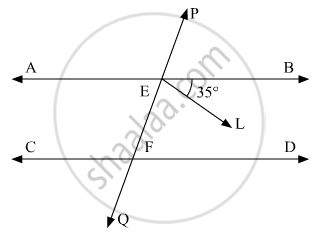# Ab and Cd Are Two Parallel Lines. Pq Cuts Ab and Cd at E and F Respectively. El is the Bisector of ∠Feb. If ∠Leb = 35°, Then ∠Cfq Will Be - Mathematics

MCQ

AB and CD are two parallel lines. PQ cuts AB and CD at E and F respectively. EL is the bisector of ∠FEB. If ∠LEB = 35°, then ∠CFQ will be

• 55°

• 70°

• 110°

• 130°

#### Solution

The figure is given as follows:It is given that, AB || CDwith PQ as transversal.

Also, EL is the bisector∠BEF and∠LEB = 35°.

We need to find∠CFQ.

Since, EL is the bisector∠BEFand ∠LEB = 35°.

Therefore,

∠BEF = 2(∠LEB)

∠BEF = 2(35°)

∠BEF = 70°       (1)

We have AB|| CD, the ∠BEF and  ∠DFE are consecutive interior angles, which must be supplementary.

∠BEF + ∠DFE = 180°

From equation (i), we get:

70° + ∠DFE  = 180°

∠DFE  = 180° - 70°

∠DFE  = 110°        ........(2)

We have and as vertically opposite angles.

Therefore,

∠CFQ = ∠DFE

∠CFQ = 110°

Concept: Parallel Lines and a Transversal
Is there an error in this question or solution?

#### APPEARS IN

RD Sharma Mathematics for Class 9
Chapter 10 Lines and Angles
Q 28 | Page 57